# PSAT Math : Other Polyhedrons

## Example Questions

### Example Question #1 : Other Polyhedrons

A regular octahedron has eight congruent faces, each of which is an equilateral triangle.

A given octahedron has edges of length five inches. Give the total surface area of the octahedron.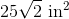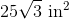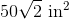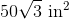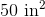Explanation:

The area of an equilateral triangle is given by the formula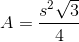Since there are eight equilateral triangles that comprise the surface of the octahedron, the total surface area is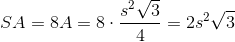Substitute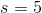: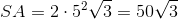square inches.

### Example Question #2 : How To Find The Surface Area Of A Polyhedron

A regular icosahedron has twenty congruent faces, each of which is an equilateral triangle.

The total surface area of a given regular icosahedron is 400 square centimeters. To the nearest tenth of a centimeter, what is the length of each edge?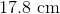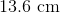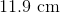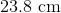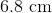Explanation:

The total surface area of the icosahedron is 400 square centimeters; since the icosahedron comprises twenty congruent faces, each has area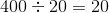square centimeters.

The area of an equilateral triangle is given by the formulaSet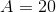and solve for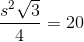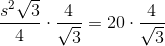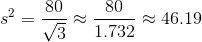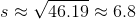centimeters.

### Example Question #1 : How To Find The Volume Of A Polyhedron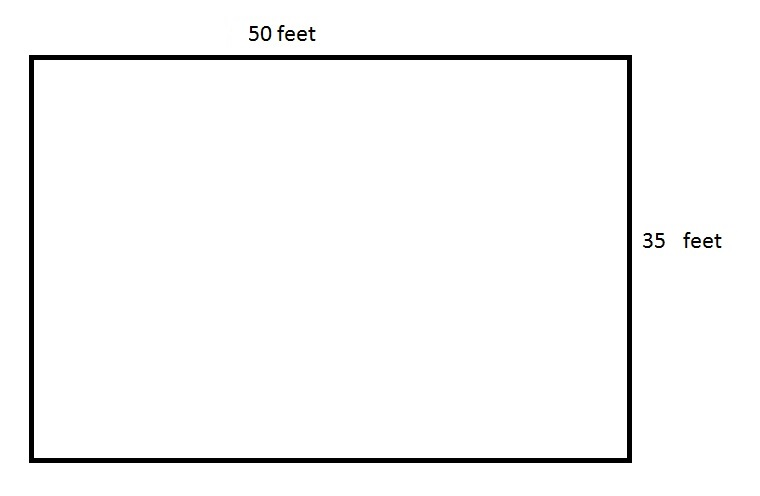The above depicts a rectangular swimming pool for an apartment. 80% of the pool is six feet deep, and the remaining part of the pool is four feet deep. How many cubic feet of water does the pool hold?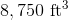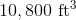None of the other choices gives the correct answer.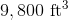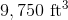Explanation:

The cross-section of the pool is the area of its surface, which is the product of its length and its width: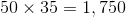square feet.

Since 80% of the pool is six feet deep, this portion of the pool holds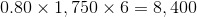cubic feet of water.

Since the remainder of the pool - 20% - is four feet deep, this portion of the pool holds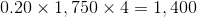cubic feet of water.

Add them together: the pool holds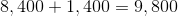cubic feet of water.